# 6.2 Numerical schemes

The fvSchemes dictionary in the system directory sets the numerical schemes for terms, such as derivatives in equations, that appear in applications being run. This section describes how to specify the schemes in the fvSchemes dictionary.

The terms that must typically be assigned a numerical scheme in fvSchemes range from derivatives, e.g. gradient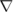, and interpolations of values from one set of points to another. The aim in OpenFOAM is to offer an unrestricted choice to the user. For example, while linear interpolation is effective in many cases, OpenFOAM offers complete freedom to choose from a wide selection of interpolation schemes for all interpolation terms.

The derivative terms further exemplify this freedom of choice. The user first has a choice of discretisation practice where standard Gaussian finite volume integration is the common choice. Gaussian integration is based on summing values on cell faces, which must be interpolated from cell centres. The user again has a completely free choice of interpolation scheme, with certain schemes being specifically designed for particular derivative terms, especially the convection divergence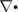terms.

The set of terms, for which numerical schemes must be specified, are subdivided within the fvSchemes dictionary into the categories listed in Table 6.2. Each keyword in Table 6.2 is the name of a sub-dictionary which contains terms of a particular type, e.g.gradSchemes contains all the gradient derivative terms such as grad(p) (which represents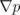). Further examples can be seen in the extract from an fvSchemes dictionary below:

 Keyword Category of mathematical terms interpolationSchemes Point-to-point interpolations of values snGradSchemes Component of gradient normal to a cell face gradSchemes Gradient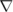divSchemes Divergence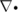laplacianSchemes Laplacian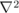timeScheme First and second time derivatives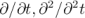Table 6.2: Main keywords used in fvSchemes.

17
18ddtSchemes
19{
20    default         Euler;
21}
22
24{
25    default         Gauss linear;
27}
28
29divSchemes
30{
31    default         none;
32    div(phi,U)      Gauss linear;
33}
34
35laplacianSchemes
36{
37    default         Gauss linear orthogonal;
38}
39
40interpolationSchemes
41{
42    default         linear;
43}
44
46{
47    default         orthogonal;
48}
49
50
51// ************************************************************************* //

The example shows that the fvSchemes dictionary comprises …Schemes sub-dictionaries containing keyword entries for each term specified within, including: a default entry; other entries whose names correspond to a word identifier for the particular term specified, e.g.grad(p) for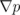If a default scheme is specified in a particular …Schemes sub-dictionary, it is assigned to all of the terms to which the sub-dictionary refers, e.g. specifying a default in gradSchemes sets the scheme for all gradient terms in the application, e.g.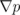,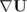. When a default is specified, it is not necessary to specify each specific term itself in that sub-dictionary, i.e. the entries for grad(p), grad(U) in this example. However, if any of these terms are included, the specified scheme overrides the default scheme for that term.

Alternatively the user may insist on no default scheme by the none entry. In this instance the user is obliged to specify all terms in that sub-dictionary individually. Setting default to none may appear superfluous since default can be overridden. However, specifying none forces the user to specify all terms individually which can be useful to remind the user which terms are actually present in the application.

The following sections describe the choice of schemes for each of the categories of terms in Table 6.2.

### 6.2.1 Interpolation schemes

The interpolationSchemes sub-dictionary contains terms that are interpolations of values typically from cell centres to face centres. A selection of interpolation schemes in OpenFOAM are listed in Table 6.3, being divided into 4 categories: 1 category of general schemes; and, 3 categories of schemes used primarily in conjunction with Gaussian discretisation of convection (divergence) terms in fluid flow, described in section 6.2.5. It is highly unlikely that the user would adopt any of the convection-specific schemes for general field interpolations in the interpolationSchemes sub-dictionary, but, as valid interpolation schemes, they are described here rather than in section 6.2.5. Note that additional schemes such as UMIST are available in OpenFOAM but only those schemes that are generally recommended are listed in Table 6.3.

A general scheme is simply specified by quoting the keyword and entry, e.g. a linear scheme is specified as default by:

default linear;

The convection-specific schemes calculate the interpolation based on the flux of the flow velocity. The specification of these schemes requires the name of the flux field on which the interpolation is based; in most OpenFOAM applications this is phi, the name commonly adopted for the surfaceScalarField velocity flux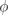. The 3 categories of convection-specific schemes are referred to in this text as: general convection; normalised variable (NV); and, total variation diminishing (TVD). With the exception of the blended scheme, the general convection and TVD schemes are specified by the scheme and flux, e.g. an upwind scheme based on a flux phi is specified as default by:

default upwind phi;

Some TVD/NVD schemes require a coefficient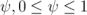where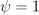corresponds to TVD conformance, usually giving best convergence and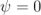corresponds to best accuracy. Running with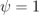is generally recommended. A limitedLinear scheme based on a flux phi with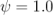is specified as default by:

default limitedLinear phi 1.0;

#### 6.2.1.1 Schemes for strictly bounded scalar fields

There are enhanced versions of some of the limited schemes for scalars that need to be strictly bounded. To bound between user-specified limits, the scheme name should be prepended by the word limited and followed by the lower and upper limits respectively. For example, to bound the vanLeer scheme strictly between -2 and 3, the user would specify:

default limitedVanLeer -2.0 3.0;
There are specialised versions of these schemes for scalar fields that are commonly bounded between 0 and 1. These are selected by adding 01 to the name of the scheme. For example, to bound the vanLeer scheme strictly between 0 and 1, the user would specify:

default vanLeer01;
Strictly bounded versions are available for the following schemes: limitedLinear, vanLeer, Gamma, limitedCubic, MUSCL and SuperBee.

#### 6.2.1.2 Schemes for vector fields

There are improved versions of some of the limited schemes for vector fields in which the limiter is formulated to take into account the direction of the field. These schemes are selected by adding V to the name of the general scheme, e.g.limitedLinearV for limitedLinear. ‘V’ versions are available for the following schemes: limitedLinearV, vanLeerV, GammaV, limitedCubicV and SFCDV.

 Centred schemes linear Linear interpolation (central differencing) cubicCorrection Cubic scheme midPoint Linear interpolation with symmetric weighting Upwinded convection schemes upwind Upwind differencing linearUpwind Linear upwind differencing skewLinear Linear with skewness correction filteredLinear2 Linear with filtering for high-frequency ringing TVD schemes limitedLinear limited linear differencing vanLeer van Leer limiter MUSCL MUSCL limiter limitedCubic Cubic limiter NVD schemes SFCD Self-filtered central differencing Gamma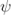Gamma differencing

Table 6.3: Interpolation schemes.

### 6.2.2 Surface normal gradient schemes

The snGradSchemes sub-dictionary contains surface normal gradient terms. A surface normal gradient is evaluated at a cell face; it is the component, normal to the face, of the gradient of values at the centres of the 2 cells that the face connects. A surface normal gradient may be specified in its own right and is also required to evaluate a Laplacian term using Gaussian integration.

The available schemes are listed in Table 6.4 and are specified by simply quoting the keyword and entry, with the exception of limited which requires a coefficient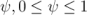where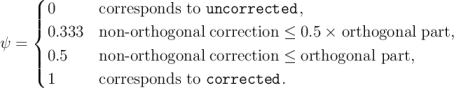(6.1)

A limited scheme with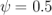is therefore specified as default by:

default limited 0.5;

 Scheme Description corrected Explicit non-orthogonal correction uncorrected No non-orthogonal correction limited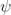Limited non-orthogonal correction bounded Bounded correction for positive scalars fourth Fourth order

Table 6.4: Surface normal gradient schemes.

The gradSchemes sub-dictionary contains gradient terms. The discretisation scheme for each term can be selected from those listed in Table 6.5.

 Discretisation scheme Description Gauss Second order, Gaussian integration leastSquares Second order, least squares fourth Fourth order, least squares cellLimited Cell limited version of one of the above schemes faceLimited Face limited version of one of the above schemes

Table 6.5: Discretisation schemes available in gradSchemes.

The discretisation scheme is sufficient to specify the scheme completely in the cases of leastSquares and fourth, e.g.

The Gauss keyword specifies the standard finite volume discretisation of Gaussian integration which requires the interpolation of values from cell centres to face centres. Therefore, the Gauss entry must be followed by the choice of interpolation scheme from Table 6.3. It would be extremely unusual to select anything other than general interpolation schemes and in most cases the linear scheme is an effective choice, e.g.

Limited versions of any of the 3 base gradient schemes — Gauss, leastSquares and fourth — can be selected by preceding the discretisation scheme by cellLimited (or faceLimited), e.g. a cell limited Gauss scheme

### 6.2.4 Laplacian schemes

The laplacianSchemes sub-dictionary contains Laplacian terms. Let us discuss the syntax of the entry in reference to a typical Laplacian term found in fluid dynamics,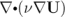, given the word identifier laplacian(nu,U). The Gauss scheme is the only choice of discretisation and requires a selection of both an interpolation scheme for the diffusion coefficient, i.e.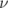in our example, and a surface normal gradient scheme, i.e.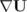. To summarise, the entries required are:

The interpolation scheme is selected from Table 6.3, the typical choices being from the general schemes and, in most cases, linear. The surface normal gradient scheme is selected from Table 6.4; the choice of scheme determines numerical behaviour as described in Table 6.6. A typical entry for our example Laplacian term would be:

laplacian(nu,U) Gauss linear corrected;

 Scheme Numerical behaviour corrected Unbounded, second order, conservative uncorrected Bounded, first order, non-conservative limited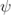Blend of corrected and uncorrected bounded First order for bounded scalars fourth Unbounded, fourth order, conservative

Table 6.6: Behaviour of surface normal schemes used in laplacianSchemes.

### 6.2.5 Divergence schemes

The divSchemes sub-dictionary contains divergence terms. Let us discuss the syntax of the entry in reference to a typical convection term found in fluid dynamics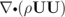, which in OpenFOAM applications is commonly given the identifier div(phi,U), where phi refers to the flux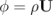.

The Gauss scheme is the only choice of discretisation and requires a selection of the interpolation scheme for the dependent field, i.e.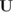in our example. To summarise, the entries required are:

Gauss <interpolationScheme>
The interpolation scheme is selected from the full range of schemes in Table 6.3, both general and convection-specific. The choice critically determines numerical behaviour as described in Table 6.7. The syntax here for specifying convection-specific interpolation schemes does not include the flux as it is already known for the particular term, i.e. for div(phi,U), we know the flux is phi so specifying it in the interpolation scheme would only invite an inconsistency. Specification of upwind interpolation in our example would therefore be:

div(phi,U) Gauss upwind;

 Scheme Numerical behaviour linear Second order, unbounded skewLinear Second order, (more) unbounded, skewness correction cubicCorrected Fourth order, unbounded upwind First order, bounded linearUpwind First/second order, bounded QUICK First/second order, bounded TVD schemes First/second order, bounded SFCD Second order, bounded NVD schemes First/second order, bounded

Table 6.7: Behaviour of interpolation schemes used in divSchemes.

### 6.2.6 Time schemes

The first time derivative (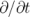) terms are specified in the ddtSchemes sub-dictionary. The discretisation scheme for each term can be selected from those listed in Table 6.8.

There is an off-centering coefficient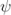with the CrankNicholson scheme that blends it with the Euler scheme. A coefficient of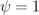corresponds to pure CrankNicholson and and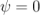corresponds to pure Euler. The blending coefficient can help to improve stability in cases where pure CrankNicholson are unstable.

 Scheme Description Euler First order, bounded, implicit localEuler Local-time step, first order, bounded, implicit CrankNicholson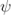Second order, bounded, implicit backward Second order, implicit steadyState Does not solve for time derivatives

Table 6.8: Discretisation schemes available in ddtSchemes.

When specifying a time scheme it must be noted that an application designed for transient problems will not necessarily run as steady-state and visa versa. For example the solution will not converge if steadyState is specified when running icoFoam, the transient, laminar incompressible flow code; rather, simpleFoam should be used for steady-state, incompressible flow.

Any second time derivative (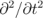) terms are specified in the d2dt2Schemes sub-dictionary. Only the Euler scheme is available for d2dt2Schemes.